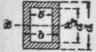The section modulus, or, as it is sometimes called, resisting inches, of a section is equal to the moment of inertia divided by the greatest distance of the neutral axis from the outside fibers of the figure or section. This rule expressed in formula would be,

Q = I / c where, Q = section modulus;

I = moment of inertia * of the section; c = greatest distance from the outside fiber to the neutral axis †. Example. - What is the section modulus for the cross-section of a 3" X 12" joist?

### Solution

Since the cross-section is rectangular, the neutral axis must pass through the center of the section, and the distance c is in this case equal to one-half the depth, that is, 6 in. The moment of inertia may be found by the method given on page 77; or more easily by the formula for rectangular sections given in Table XII, on page 83. This formula is I =bd3 / 12, where b = the breadth, and d = the depth of the joist.

Then, I = (3x12x12x12) / 12, or 432.

Having found the value of c and I, they may be substi tuted in the formula for obtaining the section modulus, and

Q = I /c = 432 / 6 = 72, which is the section modulus, or resisting inches, for a 3" X 12" joist.

Formulas for obtaining directly the section modulus the usual sections are given in Table XII, page83. The section modulus is given for the usual rolled structural sections in the tables on pages 84 to 90.

These latter are especially convenient as by them sections of different dimensions can be readily compared, and the most economical selected.

## Table XII

 Shape of Section. Moment of Inertia.I. SectionModulus.Q. Sq. LeastRadius ofGyration.R2.d4 /12 d3 /6 d2/12bd3/12 bd2/6 b2/12(b4-b'4)/12 I/.5b b2+b'2/12(bd3-b'd'3)/12 I/.5d I / Aπd4/64, or .0491d4 πd3/32,or.0982d3 d2 / 16.0491 (d4-d'4) .0982(d3-d'4/d) d2+d' 2/ 16(bd3-2b'd'3)/12 I/0.5d I / AAd2/6.66 (Approx) Ad/3.2(Approx.) I / AAd2/7.34(Approx.) Ad/3.67(Approx.) I / A

### Note

A = total area of section. In calculating the least radius of gyration be sure to use the least moment of inertia. x x' denotes the neutral axis, and the value of I given is that about this axis.